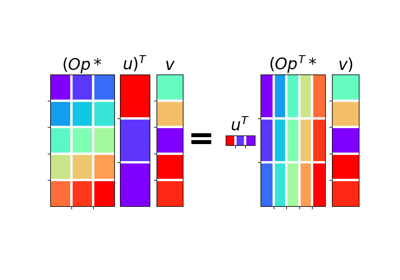# pylops.utils.dottest¶

pylops.utils.dottest(Op, nr=None, nc=None, tol=1e-06, complexflag=0, raiseerror=True, verb=False, backend='numpy')[source]

Dot test.

Generate random vectors $$\mathbf{u}$$ and $$\mathbf{v}$$ and perform dot-test to verify the validity of forward and adjoint operators. This test can help to detect errors in the operator implementation.

Parameters: Op : pylops.LinearOperator Linear operator to test. nr : int Number of rows of operator (i.e., elements in data) nc : int Number of columns of operator (i.e., elements in model) tol : float, optional Dottest tolerance complexflag : bool, optional Generate random vectors with 0: Real entries for model and data 1: Complex entries for model and real entries for data 2: Real entries for model and complex entries for data 3: Complex entries for model and data raiseerror : bool, optional Raise error or simply return False when dottest fails verb : bool, optional Verbosity backend : str, optional Backend used for dot test computations (numpy or cupy). This parameter will be used to choose how to create the random vectors. ValueError If dot-test is not verified within chosen tolerance.

Notes

A dot-test is mathematical tool used in the development of numerical linear operators.

More specifically, a correct implementation of forward and adjoint for a linear operator should verify the following equality within a numerical tolerance:

$(\mathbf{Op}\,\mathbf{u})^H\mathbf{v} = \mathbf{u}^H(\mathbf{Op}^H\mathbf{v})$

## Examples using pylops.utils.dottest¶Seislet transform02. The Dot-Test